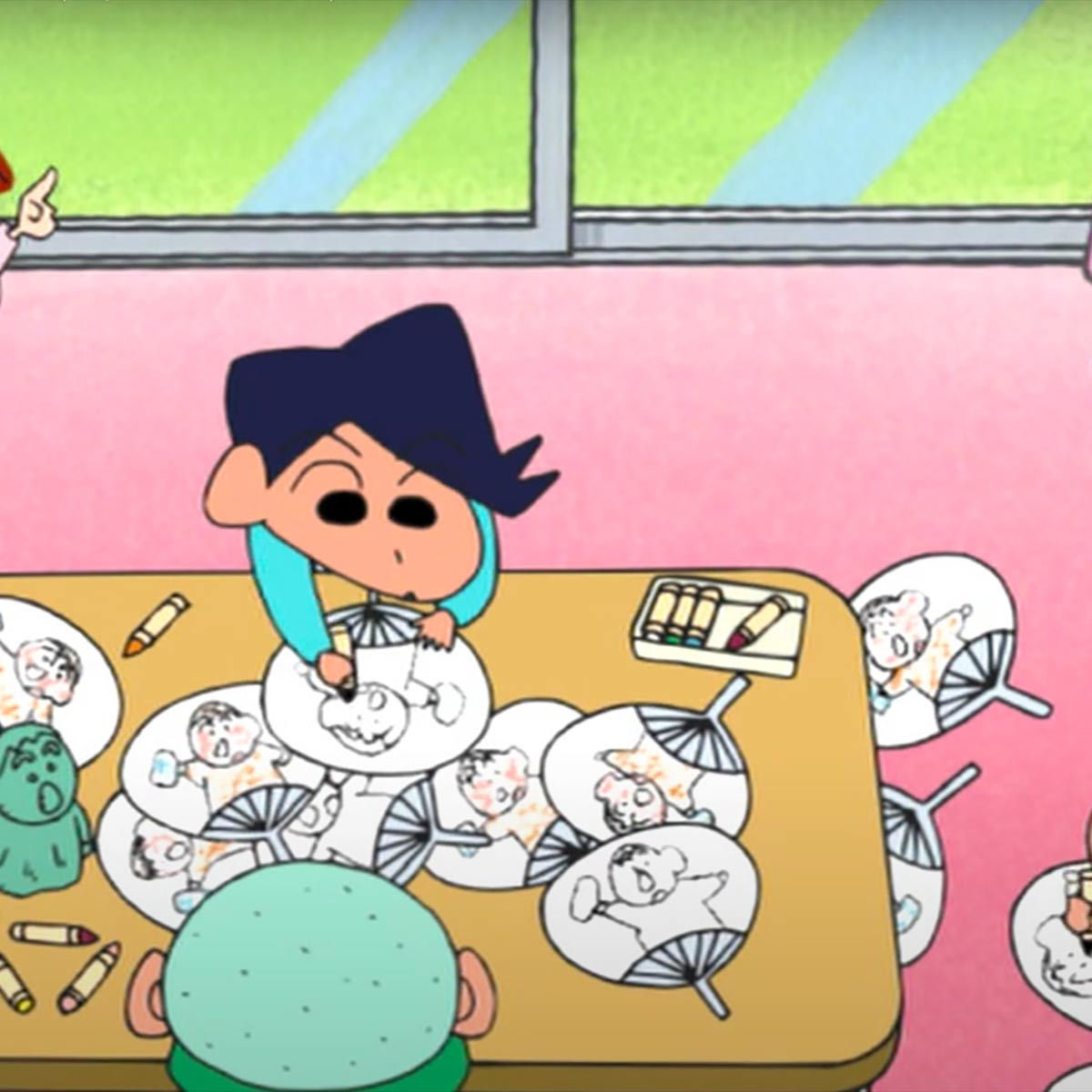# Toshiyuki Arakawa

1 Music Sheets

• ###### Birth Name:

Arakawa Toshiyuki

1953

• ###### Genres:

Toshiyuki Arakawa (Arakawa Toshiyuki), born 1953 in Japan, is a music composer, arranger and member of the Japanese Society for Rights of Authors, Composers and Publishers (JASRAC). He graduated from the Department of Literature at Chuo University and afterwards Nippon Columbia joined as music director. He currently works as a music producer, arranger and singer.

## Artist's Music Sheets

•6 0 e T Q e y 0 6 0 e T Q e y 0 6 0 e T p T [6S] 0 e T p a [TS] f [\$a] * Q e [Tp] e [9p] Q e y I G [yf] p [6S] 0 e T p T e 0 [7a] Q [rS] y f [IS] [ya] r Q [\$p] [*O] [QI] e S [TS] Q e T [9d] Q e y I f [yG] d [0a] r u p O u r 0 [6S] 0 e T p [Ta] [eS] 0 f [\$a] * Q e [Tp] Q e T [9p] Q e y I [yG] [ef] Q p [6S] 0 e T p T e 0 [7a] Q [rS] y d [*f] W [Tk] O [\$j] [*H] [QG] e f [TS] Q e T [7a] Q r y 0 [rp] [yI] 0 p [6p] 0 e T y Q [ey] u [yI] O [6p] 0 [ea] [Tp] [uS] [Tf] e 0 [pj] [9pj] Q [eOH] y [IG] [Ipj] y e Q [6pj] 0 [eak] T [SL] [pf] T e 0 [7d] [Qa] r [yS] d [*ak] r [uOH] O [\$pj] [*ak] [QOH] e [pj] [TIG] Q e T [9j] Q e [yH] G [*f] 0 e u [8H] Q [eG] Y f [7d] [Qp] e [ya] [IS] Q e [Ip] a [0S] T u T [(S] e [YG] I [TS] ( [Ip] e [7a] Q r y Q [rp] [yI] Q S [7a] Q e [yS] [Qd] [ef] [yG] [QH] [I0eyj] H G f [6S] 0 e T p [Ta] [6eS] 0 f [\$a] * Q e [Tp] Q e T [29p] Q e y I [yG] [ef] Q p [6S] 0 e T 0 e T 0 [7a] Q [rS] y f [IS] [ya] [7r] Q [\$p] [*O] [QI] e S [TS] Q e T [29d] Q e y Q [ef] [yG] Q d [30a] r u p O u r 0 [6S] 0 e T p [Ta] [eS] 0 f [\$a] * Q e [Tp] Q e T [9p] Q e y Q [eG] [yf] Q p [6S] 0 e T 0 e T 0 [I7a] Q [ypS] r [ad] [*Sf] W [Tfk] u [\$Sj] [a*H] [pQG] e [Of] [pTS] Q e T [I79eya] p i p [6p] 0 e T Q e y 0 6 0 e T Q e y p  e T 0 e T [Qe] y 0  e T 0 e T [Qe] y 0 [6e] [*T] 0
Level: 5
Intermediate